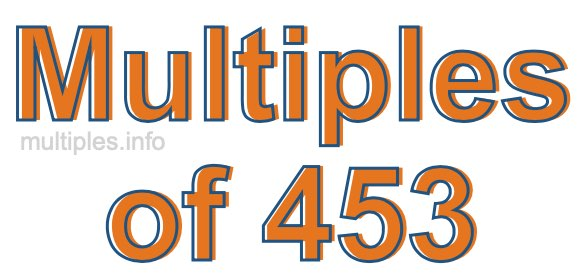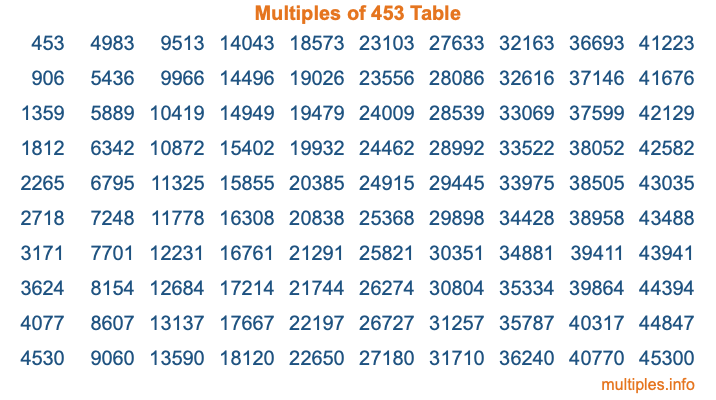Multiples of 453Welcome to the Multiples of 453 page. Here we will first teach you everything you will ever need to know about the multiples of 453, and then give you a study guide summary of everything we taught you to make sure you remember it all. Use this page to look up facts and learn information about the multiples of 453. This page will make you a multiples of four hundred fifty-three expert!

Definition of Multiples of 453
Multiples of 453 are all the numbers that when divided by 453 equal an integer. Each of the multiples of 453 are called a multiple. A multiple of 453 is created by multiplying 453 by an integer.

Therefore, to create a list of multiples of 453, you start with 1 multiplied by 453, then 2 multiplied by 453, then 3 multiplied by 453, and so on for as long as you want. Thus, the list of the first five multiples of 453 is 453, 906, 1359, 1812, and 2265. To see a larger list of multiples of 453, see the printable image of Multiples of 453 further down on this page. We also have a category where you can choose any nth multiple of 453.

Multiples of 453 Checker
The Multiples of 453 Checker below checks to see if any number of your choice is a multiple of 453. In other words, it checks to see if there is any number (integer) that when multiplied by 453 will equal your number. To do that, we divide your number by 453. If the the quotient is an integer, then your number is a multiple of 453.

Is  a multiple of 453?

Least Common Multiple of 453 and ...
A Least Common Multiple (LCM) is the lowest multiple that two or more numbers have in common. This is also called the smallest common multiple or lowest common multiple and is useful to know when you are adding our subtracting fractions. Enter one or more numbers below (453 is already entered) to find the LCM.

Check out our LCM Calculator if you need more details about the Least Common Multiple or if you need the LCM for different numbers for adding and subtraction fractions.

nth Multiple of 453
As we stated above, 453 is the first multiple of 453, 906 is the second multiple of 453, 1359 is the third multiple of 453, and so on. Enter a number below to find the nth multiple of 453.

th multiple of 453

Multiples of 453 vs Factors of 453
453 is a multiple of 453 and a factor of 453, but that is where the similarities end. All postive multiples of 453 are 453 or greater than 453. All positive factors of 453 are 453 or less than 453.

Below is the beginning list of multiples of 453 and the factors of 453 so you can compare:

Multiples of 453: 453, 906, 1359, 1812, 2265, etc.

Factors of 453: 1, 3, 151, 453

As you can see, the multiples of 453 are all the numbers that you can divide by 453 to get a whole number. The factors of 453, on the other hand, are all the whole numbers that you can multiply by another whole number to get 453.

It's also interesting to note that if a number (x) is a factor of 453, then 453 will also be a multiple of that number (x).

Multiples of 453 vs Divisors of 453
The divisors of 453 are all the integers that 453 can be divided by evenly. Below is a list of the divisors of 453.

Divisors of 453: 1, 3, 151, 453

The interesting thing to note here is that if you take any multiple of 453 and divide it by a divisor of 453, you will see that the quotient is an integer.

Multiples of 453 Table
Below is an image of the first 100 multiples of 453 in a table. The table is in chronological order, column by column. The first column has the first ten multiples of 453, the second column has the next ten multiples of 453, and so on.The Multiples of 453 Table is also referred to as the 453 Times Table or Times Table of 453. You are welcome to print out our table for your studies.

Negative Multiples of 453
Although not often discussed or needed in math, it is worth mentioning that you can make a list of negative multiples of 453 by multiplying 453 by -1, then by -2, then by -3, and so on, to get the following list of negative multiples of 453:

-453, -906, -1359, -1812, -2265, etc.

Multiples of 453 Summary
Below is a summary of important Multiples of 453 facts that we have discussed on this page. To retain the knowledge on this page, we recommend that you read through the summary and explain to yourself or a study partner why they hold true.

There are an infinite number of multiples of 453.

A multiple of 453 divided by 453 will equal a whole number.

453 divided by a factor of 453 equals a divisor of 453.

The nth multiple of 453 is n times 453.

The largest factor of 453 is equal to the first positive multiple of 453.

453 is a multiple of every factor of 453.

453 is a multiple of 453.

A multiple of 453 divided by a divisor of 453 equals an integer.

453 divided by a divisor of 453 equals a factor of 453.

Any integer times 453 will equal a multiple of 453.

Multiples of a Number
Here you can get the multiples of another number, all with the same attention to detail as we did for multiples of 453 on this page.

Multiples of
Multiples of 454
Did you find our page about multiples of four hundred fifty-three educational? Do you want more knowledge? Check out the multiples of the next number on our list!